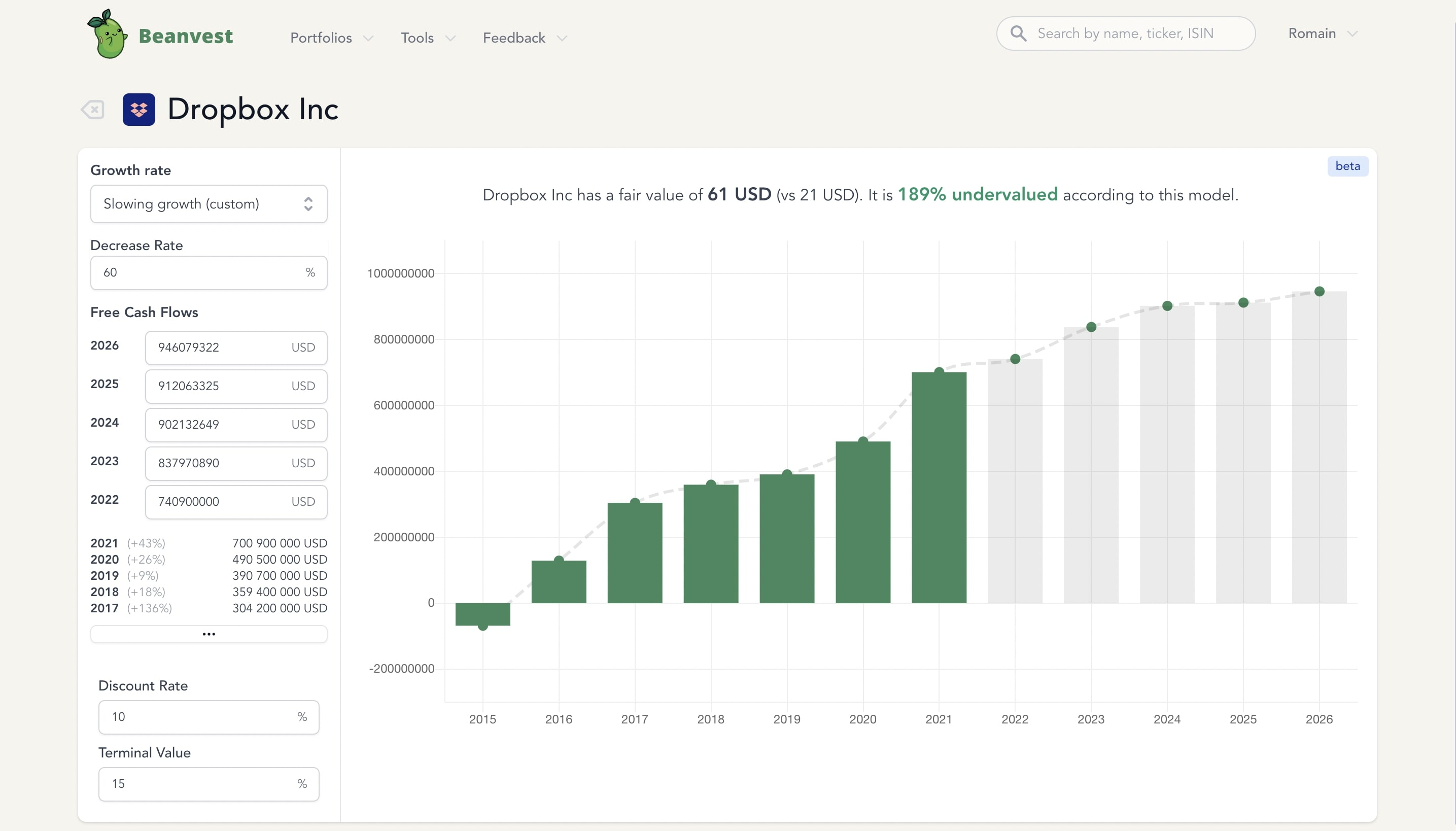# Discounted Cash Flow (DCF)

## What is a Discounted Cash Flow (DCF)?

Discounted Cash Flow (DCF) is a valuation method used to estimate the fair value of a company based on the expected future cash flows. This methods tries to estimate the present value of expected money generated in the future.

The key idea behind DCF is that $1000 in one year is worth less than$1000 now. Therefore, the value of $1000 next year must be discounted to have a lower present value. We need to use a discount factor to get the present value, which represent how much these$1000 in the future should be discounted.

For example, $1000 next year would be worth$952 in the present using a 5% discount rate.

## How to calculate DCF?

In order to calculate the present value of future cashflows, the DCF tries to actualize all the future cashflows to their present value, assuming a certain rate of return. The present value of the security is then the sum of all the future cash flows actualized to their present value.

### Discounted Cash Flow (DCF) Formula

The formula for Discounted Cash Flow (DCF) is the following :

$NPV =\sum_{i=1}^n \frac{CF_i}{(1 + R)^{i}}$

This means that the Net Present Value (NPV) of a stock is the sum of all expected future cash flows (CFi), actualized to the present value using a discount rate R.

## Example of DCF Valuation

We have estimated the following future cash flows for a company:

YearCashflow
1$1000 2$1200
3$1300 4$1500

Based on this information, we can calculate the present value of the company's future cash flows assuming we are using a 5% discount rate.

Using the formula from above, we can get the following result:

$NPV=1000\times\left(\frac{1}{(1+0.05)}\right)+1200\times\left(\frac{1}{(1+0.05)^2}\right)+1300\times\left(\frac{1}{(1+0.05)^3}\right)+1500\times\left(\frac{1}{(1+0.05)^4}\right)$

$NPV=~4231.41$

The sum of all cashflows would be $5000, but the Net Present Value (NPV) is$4231.

This reflects the fact that an amount of money in the future is worth less than the same amount today.

One of the advantages of DCF valuation is that it allows you to adjust for the time value of money. This allows investors to separate the actual value of the cash flows from the potential returns they can earn over time. This is important because it allows investors to make more informed decisions about the true value of a company.

Another advantage of using DCF for valuation is its ability to incorporate the impact of future growth. As the discount rate is applied to the future cash flows, it takes into account the expected rates of growth. This can be crucial for estimating the value of a company that is expected to grow substantially over the coming years.

The disadvantage of using DCF is that it relies heavily on expected returns and cash flows that may not materialize in the future. There is a risk that unexpected economic and market changes may drastically change the value of the company from what the analysis suggests. It also makes it difficult to accurately account for any potential risks or opportunities that the company may encounter in the future.

## What time horizon should be used for DCF?

The time horizon used for a discounted cash flow analysis should be based on the length of time in which the expected cash flows are expected to be realized. Generally, companies with longer life cycles and more predictable cash flows should use a longer time horizon, whereas companies with shorter life cycles and variable cash flows should use a shorter time horizon.

It is difficult to provide a definitive answer for what the time horizon should be for a DCF model, but generally it is recommended that analysts incorporate an appropriate time horizon for the specific company they are analyzing (e.g. 5 to 10 years). This allows analysts to incorporate a long time frame in order to better capture the long-term financial performance of the company.

However, due to the unpredicability and long-term nature of cash flows, analysts should use caution in utilizing long-term projections and ensure that the estimates are genuinely possible. You may also want to consider including an additional layer of conservatism to the model, or alternatively create a sensitivity analysis which evaluates the projected cash flows with different assumptions.

## How reliable is DCF valuation?

The reliability of a DCF model depends on the accuracy of the assumptions used. Since these models rely heavily on future projections, it means that accuracy is key when it comes to obtaining accurate results.

For this reason, it is essential that analysts employ rigorous methods to determine the expected cash flows and discount rate. They should utilise up to date information when allowing for expense growth, inflation, taxes, and other potential economic changes. Moreover, analysts should also give extra thought to how their results will be impacted by the riskiness of the asset. This will help them determine an appropriate discount rate and decide if a higher or lower discount rate is more prudent based on the level of associated risk.

Overall, the accuracy of any DCF model will heavily rely on the inputs that are used and, as a result, this method may not always be the most reliable tool for determining a security's fair value. However, despite its flaws it is a very useful tool for understanding how the future cash flows generated by a company will impact its value.

## DCF Calculator

Beanvest has a built-in DCF Calculator that will help you make the DCF calculation easily for stocks.The discounted cash flow calculator saves you time because it automatically pulls data for Free Cash Flows from previous years. You can select a cash flow growth scenario, and the tools will make the projections and automatically calculate the fair value of the stock you selected.

You can create an account below to use this free DCF calculator.

## Ready to Become a Better Investor?

14-day Premium trial, 100% free after.